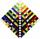# Sequence 11

What is the nth term of this sequence 1,1/2,1/3,1/4,1/5 ?

Result

x = (Correct answer is: 1/n)#### Solution:Leave us a comment of example and its solution (i.e. if it is still somewhat unclear...):

Showing 0 comments:Be the first to comment!#### To solve this example are needed these knowledge from mathematics:

Looking for help with calculating harmonic mean? Looking for a statistical calculator?

## Next similar examples:

1. TreesA certain species of tree grows an average of 0.5 cm per week. Write an equation for the sequence that represents the weekly height of this tree in centimeters if the measurements begin when the tree is 200 centimeters tall.
2. CoefficientDetermine the coefficient of this sequence: 7.2; 2.4; 0.8
3. Six termsFind the first six terms of the sequence a1 = -3, an = 2 * an-1
4. SequenceWrite the first 6 members of these sequence: a1 = 5 a2 = 7 an+2 = an+1 +2 an
5. AP - simpleDetermine the first nine elements of sequence if a10 = -1 and d = 4
6. Sequence 3Write the first 5 members of an arithmetic sequence: a4=-35, a11=-105.
7. SequenceWrite the first 7 members of an arithmetic sequence: a1=-3, d=6.
8. Sequence 2Write the first 5 members of an arithmetic sequence a11=-14, d=-1
9. AS sequenceIn an arithmetic sequence is given the difference d = -3 and a71 = 455. a) Determine the value of a62 b) Determine the sum of 71 members.
10. SequenceBetween numbers 1 and 53 insert n members of the arithmetic sequence that its sum is 702.
11. Nineteenth memberFind the nineteenth member of the arithmetic sequence: a1=33 d=5 find a19
12. AP - simpleFind the first ten members of the sequence if a11 = 132, d = 3.
13. Geometric progression 2There is geometric sequence with a1=5.7 and quotient q=-2.5. Calculate a17.
14. Sum of membersWhat is the sum of the first two members of the aritmetic progression if d = -4.3 and a3 = 7.5?
15. Theorem proveWe want to prove the sentense: If the natural number n is divisible by six, then n is divisible by three. From what assumption we started?
16. SeatsSeats in the sport hall are organized so that each subsequent row has five more seats. First has 10 seats. How many seats are: a) in the eighth row b) in the eighteenth row
17. SeriesYour task is express the sum of the following arithmetic series for n = 14: S(n) = 11 + 13 + 15 + 17 + ... + 2n+9 + 2n+11